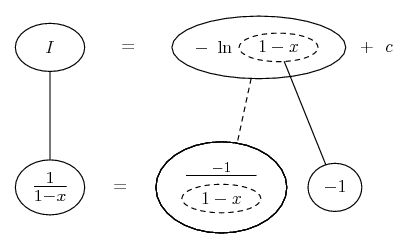# Thread: integral of 1/ (1-x)

1. ## integral of 1/ (1-x)

is the answer to this integral, 1/ (1-x)

= ln l 1 - x l

or

= - ln l 1 - x l

if its - ln l1-x l , could you explain why.

thanks.

2. Differentiate your second (correct) version, applying the chain rule.

3. Hello, johntuan!

Is the answer to this integral: .$\displaystyle \int\frac{dx}{1-x}$

. . $\displaystyle \ln|1 - x| + C\;\text{ or }\;-\ln |1-x| + C$ ?

If it is $\displaystyle -\ln |1-x|+C$, could you explain why?

We have: .$\displaystyle \int\frac{dx}{1-x}$

Let: .$\displaystyle u \:=\:1-x\quad\Rightarrow\quad du \:=\:{\color{red}-}dx \quad\Rightarrow\quad dx \:=\:{\color{red}-}du$

Substitute: .$\displaystyle \int\frac{{\color{red}-}du}{u} \;=\;{\color{red}-}\int\frac{du}{u} \;=\;{\color{red}-}\ln|u| + C$

Back-substitute: .$\displaystyle {\color{red}-}\ln|1-x| + C$

4. Just in case a picture helps...Straight continuous lines differentiate downwards (integrate up) with respect to x, the straight dashed line similarly but with respect to the dashed balloon expression, so that the triangular network, on the right, satisfies the chain rule.

Don't integrate - balloontegrate!

Balloon Calculus Forum

#### Search Tags

1 or, integral